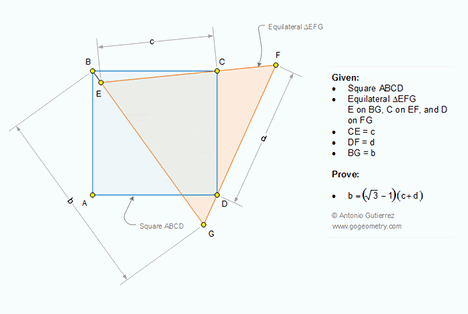## Saturday, July 23, 2016

### Geometry Problem 1238: Square, Equilateral Triangle, Metric Relations. Mobile Apps

Geometry Problem. Post your solution in the comment box below.
Level: Mathematics Education, High School, Honors Geometry, College.

Click the figure below to view more details of problem 1238.#### 1 comment:

1.https://goo.gl/photos/JZ6HdcxZpLijf4a98

Let x= CF
Locate point H such that CH=CF= x and CH ⊥EF ( see sketch)
Note that triangle CDF is the image of CBH in the rotation transformation centered C , rotation angle= 90
So BH= FD= d and BH⊥DF
BPG is 30-60-90 triangle and FHP is 15-75-90 triangle
We have BP= BH+HP = b. √3/2….. (1)
Replace HP= FH.sin 15= x(√3-1)/2 and BH= d in (1) we will get
3.b= 2√3. d + (3-√3)x …. (2)
Since EFG is equilateral so EF=EC+EF=FP+PG… (3)
Replace FP= GH.cos15=x.( √3+1)/2 and PG= b/2 in (3) we will get
2(x+c)=b+ x(√3+1)….. ( 4)
Eliminate x from (2) and (4) we will get b= (√3-1)(c+d)